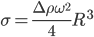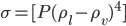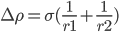# thesIt

•#### Arif 12:06:03 pm on February 19, 2010 | 0 | # | Tags: angular velocity, density, equation, interfacial tension, spinning drop, wikipedia

Interfacial tension and centrifugal forces balanced at mechanical equilibrium.centrifugal-IFT equilibrium

Interfacial tension of any liquid that gives a shape very close to a cylinder at the equilibrium point, can be estimated using Vonnegut’s expression:$\sigma=\frac{\Delta\rho\omega^2}{4}R^3$

ω : angular velocity; (radians per second, degrees per second, revolutions per second)

Δρ : difference between two fluid: the less-dense and the dense

• #### Arif 06:19:05 pm on January 1, 2010 | 0 | # | Tags: density, equation, Escobedo 1998, interfacial tension, Kumar 2005, parachor, prediction, pure compound, reference, statistical

Escobedo and Mansoori (AIChE J. 42(5): 1425, 1996) Found original paper: check Escobedo 1996

The proposed 1996 paper relates the surface tension of mixtures to bulk-phase concentrations and properties (i.e. densities). Surface tension of pure organic fluids σ:$\sigma=[P(\rho_l-\rho_v)^4]$

The two ρ are densities: liquid and vapour.

They introduced parachor (P), a new expression, derived from statistical mechanics (from Macleod equation) which represents the experimental surface tension of 94 different organic compounds within 1.05 AAD% (average absolute deviations).

(Kumar 2005) It expresses the surface tension of a liquid in equilibrium with its own vapour. Historical reference for parachor cited here: Macleod, Sugden, Quayle, Escobedo.

• #### Arif 04:57:56 pm on January 1, 2010 | 0 | # | Tags: basic, equation, interfacial tension, Myers 2006, Pashley 2004

Young Laplace equation:$\Delta\rho=\sigma(\frac{1}{r1}+\frac{1}{r2})$

other uses γ instead of σ for surface/interfacial tension (i.e. Pashley 2004). Common unit is dyne/cm (1 dyne = 1 micro Newton (μN) ).